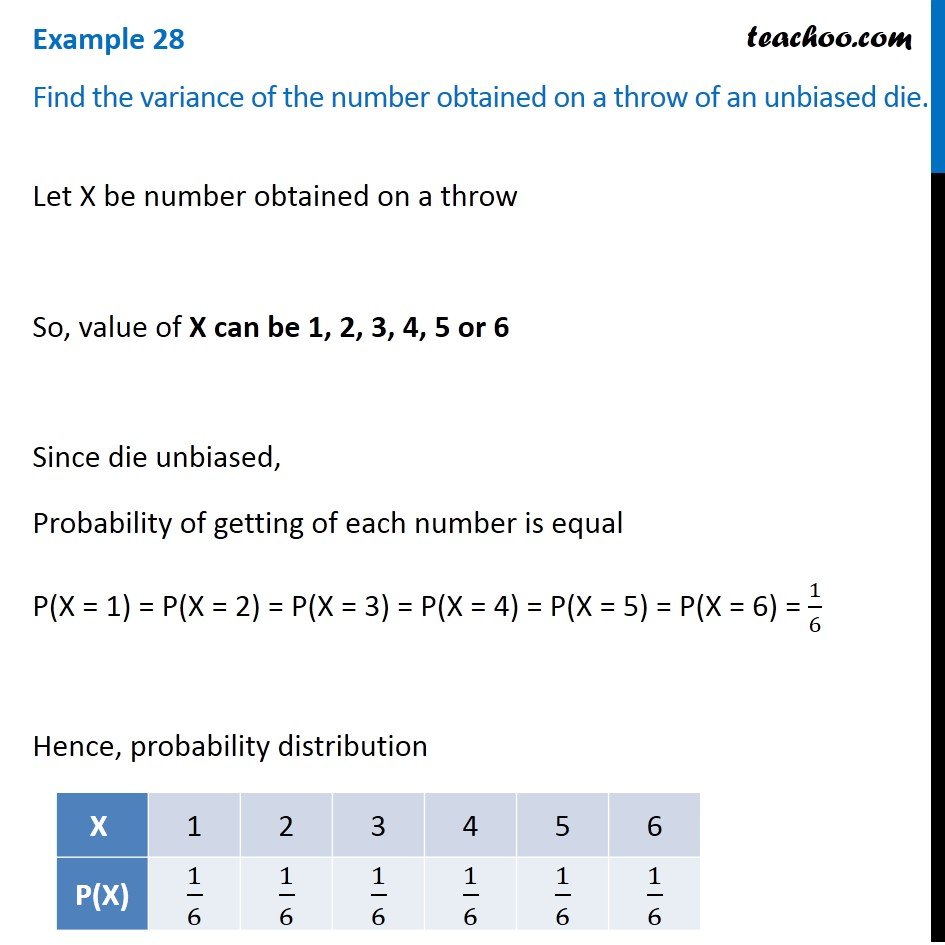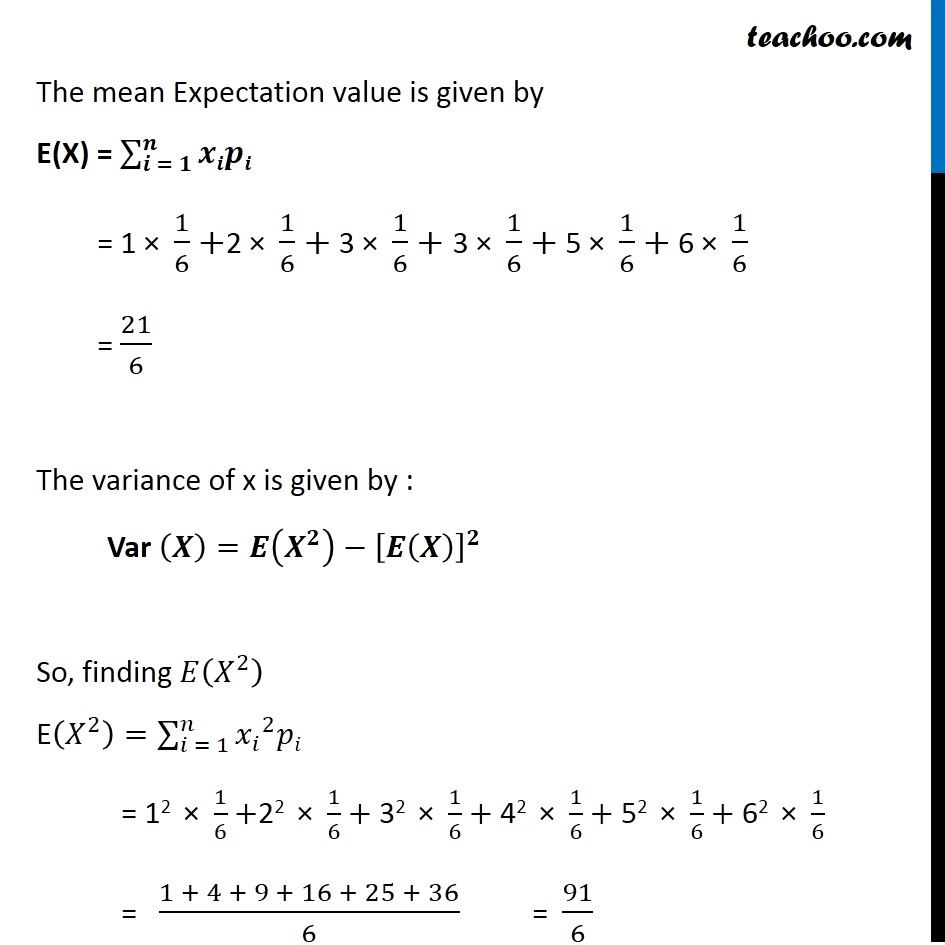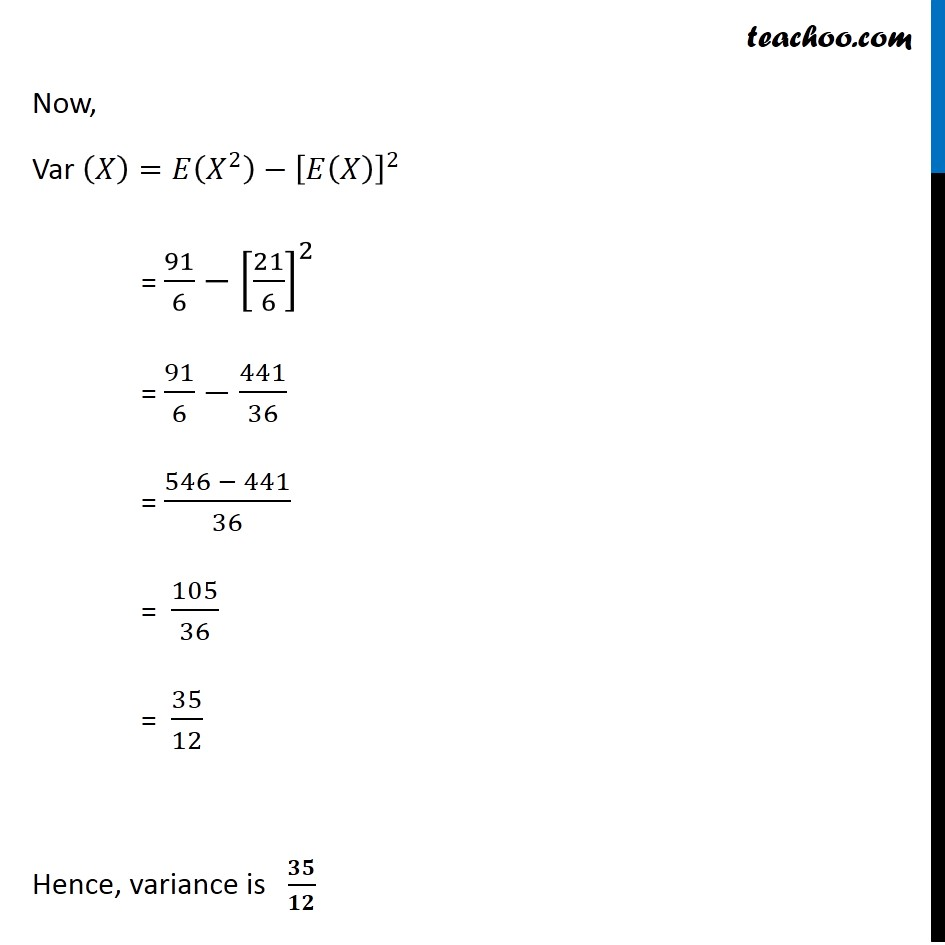Chapter 13 Class 12 Probability

Class 12
Important Questions for exams Class 12Learn in your speed, with individual attention - Teachoo Maths 1-on-1 Class

### Transcript

Question 7 Find the variance of the number obtained on a throw of an unbiased die. Let X be number obtained on a throw So, value of X can be 1, 2, 3, 4, 5 or 6 Since die unbiased, Probability of getting of each number is equal P(X = 1) = P(X = 2) = P(X = 3) = P(X = 4) = P(X = 5) = P(X = 6) = 1/6 Hence, probability distribution The mean Expectation value is given by E(X) = ∑2_(𝒊 = 𝟏)^𝒏▒𝒙𝒊𝒑𝒊 = 1 × 1/6+2 × 1/6+ 3 × 1/6+ 3 × 1/6+ 5 × 1/6+ 6 × 1/6 = 21/6 The variance of x is given by : Var (𝑿)=𝑬(𝑿^𝟐 )−[𝑬(𝑿)]^𝟐 So, finding 𝐸(𝑋^2 ) E(𝑋^2 )=∑2_(𝑖 = 1)^𝑛▒〖〖𝑥_𝑖〗^2 𝑝𝑖〗 = 12 × 1/6+22 × 1/6+ 32 × 1/6+ 42 × 1/6+ 52 × 1/6+ 62 × 1/6 = (1 + 4 + 9 + 16 + 25 + 36)/6 = 91/6 Now, Var (𝑋)=𝐸(𝑋^2 )−[𝐸(𝑋)]^2 = 91/6−[21/6]^2 = 91/6−441/36 = (546 − 441)/36 = 105/36 = 35/12 Hence, variance is 𝟑𝟓/𝟏𝟐